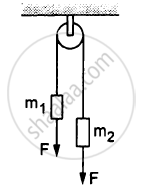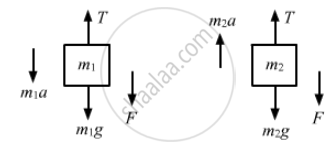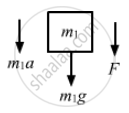# In the Following Figure , M1 = 5 Kg, M2 = 2 Kg and F = 1 N. Find the Acceleration of Either Block. Describe the Motion of M1 If the String Breaks but F Continues to Act. - Physics

Sum

In the following figure, m1 = 5 kg, m2 = 2 kg and F = 1 N. Find the acceleration of either block. Describe the motion of m1 if the string breaks but F continues to act.#### Solution

Let the acceleration of the blocks be a.
The free-body diagrams for both the blocks are shown below:From the free-body diagram,
m1a = m1g + F − T    ...(i)

Again, from the free-body diagram,
m2a = T − m2g − F    ...(ii)

Adding equations (i) and (ii), we have:
$a = g\frac{m_1 - m_2}{m_1 + m_2}$
$\Rightarrow a = \frac{3g}{7} = \frac{29 . 4}{7}$
$= 4 . 2 m/ s^2$

Hence, acceleration of the block is 4.2 m/s2.
After the string breaks, m1 moves downward with force F acting downward. Then,m1a = F + m1g
5a = 1 + 5g
$\Rightarrow a = \frac{5g + 1}{5}$
$= g + 0 . 2 m/ s^2$
Concept: Newton’s Second Law of Motion
Is there an error in this question or solution?

#### APPEARS IN

HC Verma Class 11, Class 12 Concepts of Physics Vol. 1
Chapter 5 Newton's Laws of Motion
Q 27 | Page 81